Processing ......FreeComputerBooks.com Links to Free Computer, Mathematics, Technical Books all over the World

Automata Theory: An Algorithmic Approach
🌠 Top Free Unix/Linux Books - 100% Free or Open Source!
• Title Automata Theory: An Algorithmic Approach
• Author(s) Javier Esparza and Michael Blondin
• Publisher: The MIT Press (October 17, 2023); eBook (Draft, Creative Commons Licensed)
• Paperback 560 pages
• eBook PDF (Draft, 321 pages)
• Language: English
• ISBN-10: 0262048639
• ISBN-13: 978-0262048637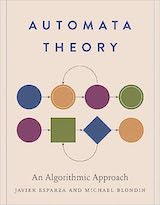Book Description

A comprehensive introduction to Automata Theory that uses the novel approach of viewing automata as data structures.

This textbook presents automata theory from a fresh viewpoint inspired by its main modern application, program verification, where automata are viewed as data structures for the algorithmic manipulation of sets and relations.

• Uses novel “automata as data structures” approach
• Algorithm approach ideal for programmers looking to broaden their skill set and researchers in automata theory and formal verification
• The first introduction to automata on infinite words that does not assume prior knowledge of finite automata
• Thorough, engaging presentation of concepts balances description, examples, and theoretical results
• Extensive illustrations, exercises, and solutions deepen comprehension
• Javier Esparza is Professor and Chair of Foundations of Software Reliability and Theoretical Computer Science at the Technical University of Munich and coauthor of Free Choice Petri Nets and Unfoldings: A Partial-Order Approach to Model Checking.
• Michael Blondin is Associate Professor of Computer Science at the Université de Sherbrooke.
Reviews, Rating, and Recommendations: Related Book Categories: Read and Download Links:Similar Books:
•Logic and Automata: History and Perspectives (Jorg Flum, et al)

This book gives a consolidated overview of the research results achieved in the theory of automata and logic, covers many different facets of logic and automata theory, emphasizing the connections to other disciplines such as games, algorithms, etc.

•A Friendly Introduction to Mathematical Logic (Chris Leary)

In this user-friendly book, readers with no previous study in the field are introduced to the basics of model theory, proof theory, and computability theory, leading to rigorous proofs of Gödel's First and Second Incompleteness Theorems.

•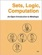Sets, Logic, Computation: An Open Introduction to Metalogic

It covers naive set theory, first-order logic, sequent calculus and natural deduction, the completeness, compactness, and Löwenheim-Skolem theorems, Turing machines, and the undecidability of the halting problem and of first-order logic.

•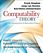Computability Theory: Introduction to Recursion Theory

Computability Theory originated with the seminal work of Gödel, Church, Turing, Kleene and Post in the 1930s. These texts provide concise, comprehensive, and authoritative introduction to contemporary computability theory, techniques, and results.

•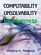Computability, Unsolvability, Randomness (Stephen G. Simpson)

The author exposits Turing's 1936 theory of computability and unsolvability, as subsequently developed by Kleene and Post. This theory is of the essence in theoretical computer science and in the study of unsolvable mathematical problems.

•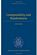Computability and Randomness (Andre Nies)

The complexity and the randomness aspect of a set of natural numbers are closely related. This book includes a detailed treatment of Turing's theory of computability and unsolvability as subsequently developed by Kleene, Post, Friedberg, etc.

•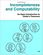Incompleteness and Computability: Gödel's Theorems

This book is an introduction to metamathematics and Gödel's Theorems. It covers recursive function theory, arithmetization of syntax, the first and second incompleteness theorem, models of arithmetic, second-order logic, and the lambda calculus.

•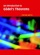An Introduction to Gödel’s Theorems (Peter Smith)

The formal explanations are interwoven with discussions of the wider significance of the two Gödel's Theorems. It will be of equal interest to mathematics students taking a first course in mathematical logic.

•What is Mathematics: Godel's Theorem and Around (K. Podnieks)

This accessible book gives a new, detailed and elementary explanation of the Gödel's Incompleteness Theorems and presents the Chaitin results and their relation to the da Costa-Doria results, which are given in full, but with no technicalities.

•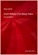Gödel Without (Too Many) Tears (Peter Smith)

How is this remarkable result of Gödel's Theorems established? This short book explains. The aim is to make the Theorems available, clearly and accessibly, even to those with a quite limited formal background.

•Introduction to Mathematical Logic (Vilnis Detlovs, et al)

This book explores the principal topics of mathematical logic. It covers propositional logic, first-order logic, first-order number theory, axiomatic set theory, and the theory of computability. Discusses the major results of Gödel, Church, Kleene, Rosser, and Turing.

•Introduction to Theory of Computation (Anil Maheshwari, et al)

This is a textbook on formal languages, automata theory, and computational complexity. This text strikes a good balance between rigor and an intuitive approach to computer theory.

Book Categories
 :All CategoriesTop Free BooksRecent BooksMiscellaneous BooksComputer EngineeringComputer LanguagesComputer ScienceData Science/DatabasesJava and Java EE (J2EE)Linux and UnixMathematicsMicrosoft and .NETMobile ComputingNetworking and CommunicationsSoftware EngineeringSpecial TopicsWeb Programming
Other Categories